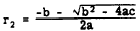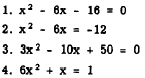Custom SearchTHE DISCRIMINANT The roots of a quadratic equation may be classified in accordance with the following criteria: 1. Real or imaginary. 2. Rational or irrational.   3. EquaI or unequal. The task of discriminating among these possible characteristics to find the nature of the roots is best accomplished with the aid of the quadratic formula. The part of the quadratic formula which is used is called the DISCRIMINANT. If the roots of a quadratic are denoted by the symbols r1 and r2, then the following relations may be stated:We can show that the character of the roots is dependent upon the form taken by the expression b2 - 4ac which is the quantity under the radical in the formula. This expression is the DISCIUMINANT of a quadratic equation. IMAGINARY ROOTS Since there is a radical in each root, there is a possibility that the roots could be imaginary. They are imaginary when the number under the radical in the quadratic formula is negative (less than 0). In other words, when the value of the discriminant Is less than 0, the roots are imaginary. EXAMPLE:Thus, without further work, we know that the roots are imaginary. CHECK: The roots areWe recognize both of these numbers as being imaginary. We may also conclude that when one root is imaginary the other will also be imaginary. This is because the pairs of imaginary roots are always conjugate complex numbers. If one root is of the form a + ib, then a - ib is also a root. Knowing that imaginary roots always occur in pairs, we can conclude that a quadratic equation always has either two imaginary roots or two real roots. Practice problems. Using the discriminant, state whether the roots of the following equations are real or imaginary:Answers: 1. Real 2. Imaginary 3. Imaginary 4. Real